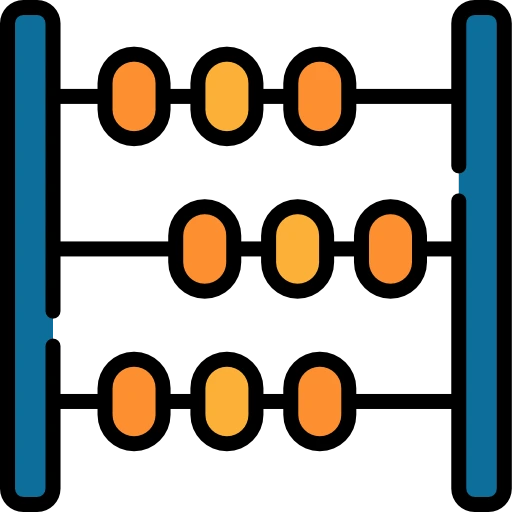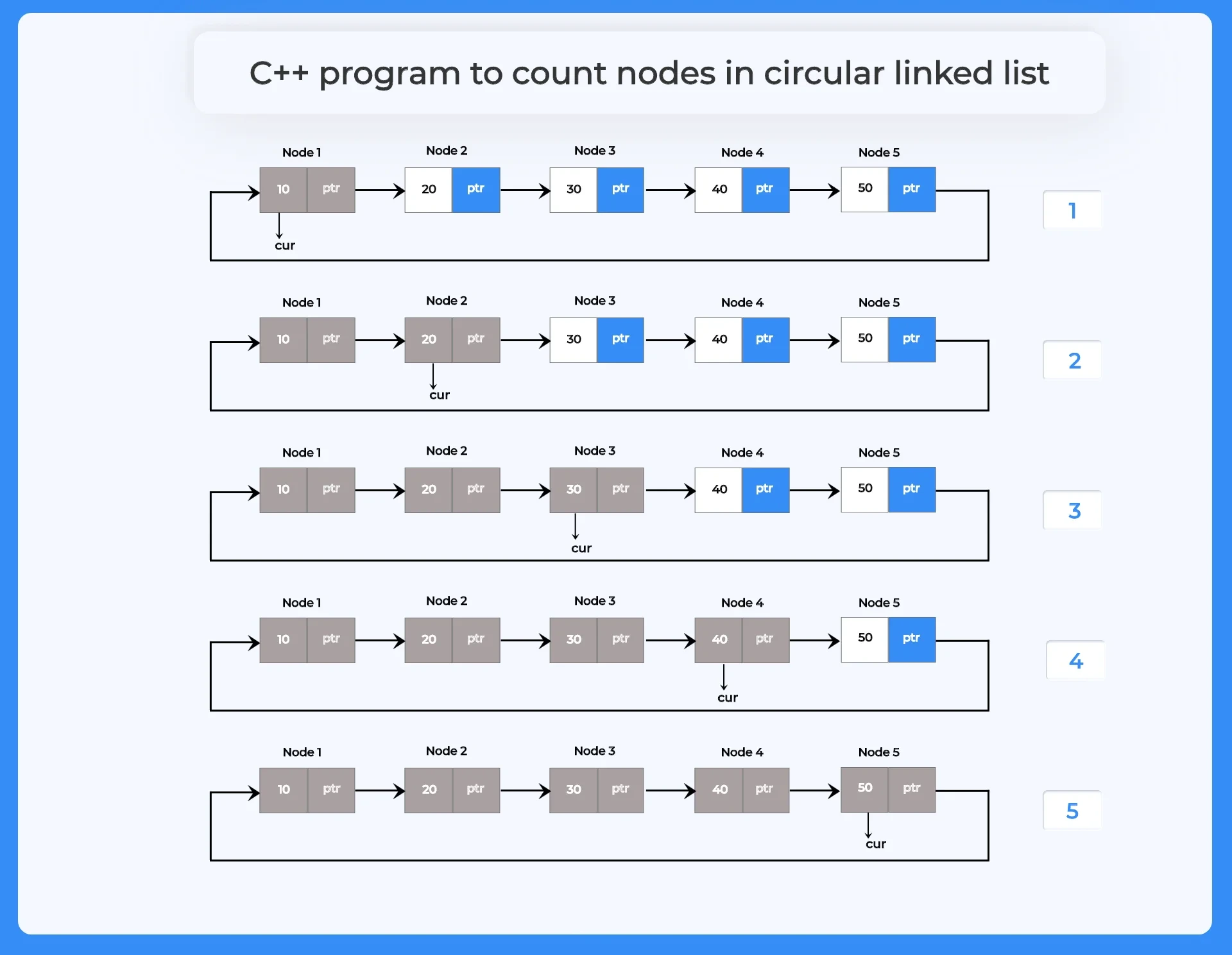# C++ program to count nodes in circular linked list

## How to count nodes in circular linked list in C++?

To write a C++ program to count nodes in circular linked list, we need to initialize a node pointer and a variable that will store the count of nodes. Use the node pointer to point each node of the list and increase the count of the counter variable by one on every successful iteration. Stop the iteration when node pointer points the head.## Steps write a C++ program to count nodes in circular linked list

1. Write set of codes to construct circular linked list.
2. Create a function to build the circular linked list.
3. Make a function to print the circular linked list data.
4. Create a function to count the number of nodes present in circular linked list.
5. In this function initialize a variable to count the number of nodes.
6. Initialize a pointer that will point to each node on every successful iteration.
7. Stop the iteration when pointer points the head again.
8. Return the count of nodes.
9. Now call these functions in the main function and print the total count of nodes.
```struct Node
{
int num;
struct Node *next;
};```## Algorithm to count nodes in circular linked list in C++

• INT CNT=0
• STRUCT NODE CUR = HEAD
• DO
• CUR = CUR -> NEXT
• CNT++
• RETURN CNT

## C++ program for counting nodes in circular linked list

Run
```#include<iostream>
using namespace std;

struct Node
{
int num;
struct Node *next;

void insertStart (struct Node **head, int data)	//function to create linked list
{
struct Node *newNode = (struct Node *) malloc (sizeof (struct Node));
newNode->num = data;

// if its the first node being entered
{

cout << newNode->num << " Inserted\n";
return;
}

// if CLL already as >=1 node

// traverse till last node in CLL
{
curr = curr->next;
}

curr->next = newNode;		// last node's next as this new node

cout << newNode->num << " Inserted\n";

// previous head node becomes 2nd node
}

{

//cout << "\nCircular Linked List : " << endl;

// if circular linked list is empty currently
return;

// since we need to take care of circular nature of linked list
do
{
cout << temp->num << " ";
temp = temp->next;

}
cout << endl;
}

int count (struct Node *head)	//function to count number of nodes
{
int cnt = 0;

//Iterating till end of list
do
{
cur = cur->next;
cnt++;
}

return cnt;
}

int main ()				//main function
{

cout << "\nCircular linked list data:\n";
cout << "\nTotal number of nodes are: " << count (head);
}
```
```Output:
1 Inserted
2 Inserted
3 Inserted
4 Inserted
5 Inserted

5 4 3 2 1

Total number of nodes are: 5```

### Related Banners

Get PrepInsta Prime & get Access to all 200+ courses offered by PrepInsta in One Subscription

## Get over 200+ course One Subscription

Courses like AI/ML, Cloud Computing, Ethical Hacking, C, C++, Java, Python, DSA (All Languages), Competitive Coding (All Languages), TCS, Infosys, Wipro, Amazon, DBMS, SQL and others

## Checkout list of all the video courses in PrepInsta Prime Subscription

• Introduction to Circular Linked List
• Circular Linked List in –
C | C++ | Java
• Insertion in Circular Linked List –
C | C++ | Java
• Insertion at the beginning–
C | C++ | Java
• Insertion at the end –
C | C++ | Java
• Insertion at nth position –
C | C++ | Java
• Deletion in Circular Linked List –
C | C++ | Java
• Deletion from beginning in Circular Linked List –
C | C++ | Java
• Deletion from nth position in Circular Linked List –
• Deletion from end in Circular Linked List –
C | C++ | Java
• Insertion and Deletion in Circular Linked List – C | C++ | Java
• Split a Circular Linked List in two halves –
C | C++ | Java
• Count nodes in Circular Linked List –
C | C++ | Java
• Sorted Insert In Circular Linked List –
C | C++ | Java
• Insertion in the middle in Circular Linked List –
C | C++ | Java